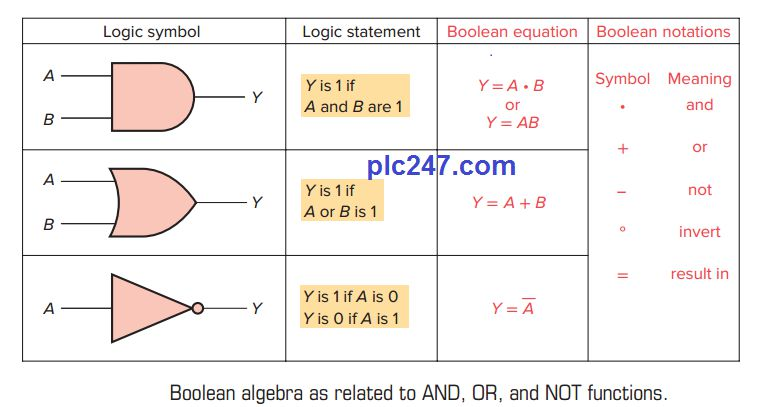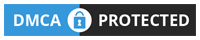PLC Guides

# Fundamentals of LogicWritten by

#### 1. The Binary Concept

The PLC, like all digital equipment, operates on the binary principle. The term binary principle refers to the idea that many things can be thought of as existing in only one of two states. These states are 1 and 0. The 1 and 0 can represent ON or OFF, open or closed, true or false, high or low, or any other two conditions. The key to the speed and accuracy with which binary information can be processed is that there are only two states, each of which is distinctly different. There is no in-between state so when information is processed the outcome is either yes or no.
A logic gate is a circuit with several inputs but only one output that is activated by particular combinations of input conditions. The two-state binary concept, applied
to gates, can be the basis for making decisions.

#### 2. The AND Function

An AND gate is a device with two or more inputs and one output. The AND gate output is 1 only if all inputs are 1.

The basic rules that apply to an AND gate are:

+ If all inputs are 1, the output will be 1.

+ If any input is 0, the output will be 0.

#### 2. The OR Function

The OR gate output is 1 if one or more inputs are 1.

The basic rules that apply to an OR gate are:

+ If one or more inputs are 1, the output is 1.

+ If all inputs are 0, the output will be 0.

#### 3. The NOT Function

Unlike the AND and OR functions, the NOT function can have only one input. The NOT output is 1 if the input is 0. The output is 0 if the input is 1. The result of the NOT operation is always the inverse of the input, and the NOT function is, therefore, called an inverter. The NOT function is often depicted by using a bar across the top of the letter, indicating an inverted output. The small circle at the output of the inverter is referred to as a bubble and indicates that an inversion of the logical function has taken place.

The NOT symbol placed at the output of an AND gate would invert the normal output result. An AND gate with an inverted output is called a NAND gate. The NAND
gate symbol and truth table are shown in Figure below. The NAND function is often used in integrated circuit logic arrays and can be used in programmable controllers to solve complex logic.

The same rule about inverting the normal output result applies if a NOT symbol is placed at the output of the OR gate. The normal output is inverted, and the function is referred to as a NOR gate.

#### 4. The Exclusive-OR (XOR) Function

An often-used combination of gates is the exclusive-OR
(XOR) function. The XOR gate symbol and truth table are shown in Figure below. The output of this circuit is HIGH only when one input or the other is HIGH, but not both.
The exclusive-OR gate is commonly used for the comparison of two binary numbers.

#### 5. Boolean Algebra

The mathematical study of the binary number system and logic is called Boolean algebra. The purpose of this algebra is to provide a simple way of writing complicated combinations of logic statements. There are many applications where Boolean algebra could be applied to solving PLC programming problems.
Here are some examples to solve problems with Boolean Algebra